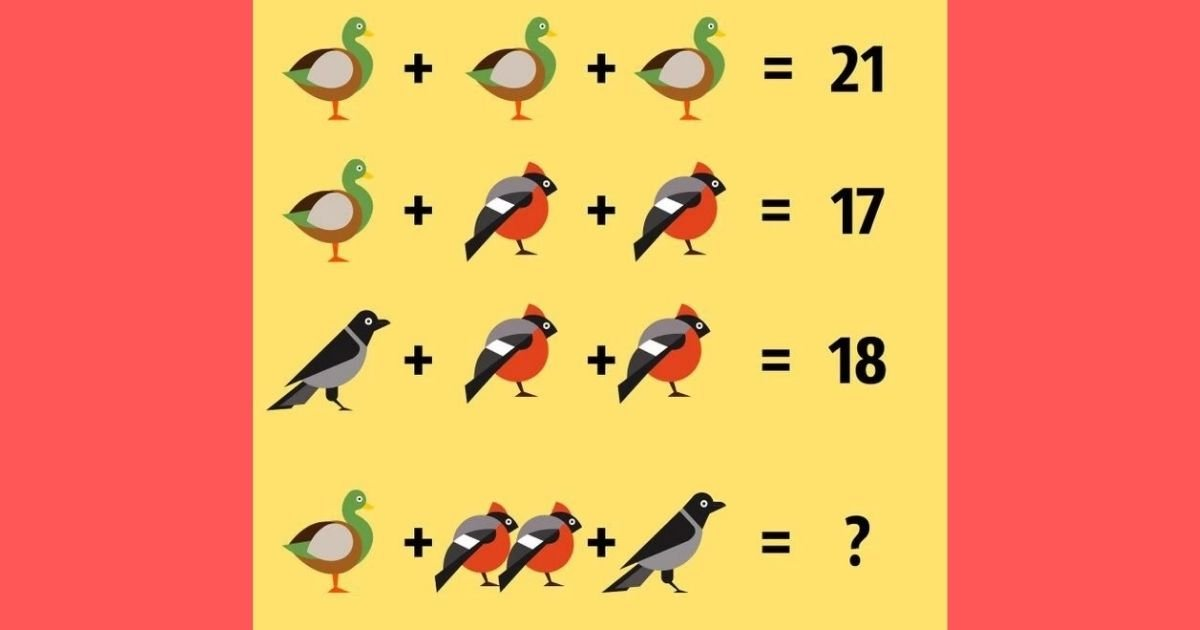# Most People Can’t Solve This Children’s Puzzle! But Can You?

Older generations were perplexed by a math puzzle for youngsters that was popular on social media.

If you haven’t trained your arithmetic abilities in a long time, now is the time to give your brain cells something to ponder about.Take a close look at the image above and tell us what you think the solution is. As you can see, there are a variety of birds strewn across four lines that resemble four distinct equations.

Your task is to figure out which number should replace the question mark at the bottom using the first three lines.

Before we release the answer, here are some pointers that may assist you in solving the puzzle on your own. Between 20 and 30 is the correct answer. The solution is an odd number as well!

Do you have any suggestions yet? Share your final answer with your friends on Facebook, and don’t forget to dare them to solve the problem as well.

This arithmetic problem’s solution is 25, and here’s how we arrived at that figure.

Let’s pretend that D stands for the duck, R for the red bird, and B for the black bird in order to illustrate the answer mathematically.

LINE 1: D + D + D = 21. Therefore, 3D = 21 and D = 7. The value of a duck is 7.

LINE 2: D + R + R = 17. Hence, 7 + 2R = 17 and 2R = 10. Therefore, R = 5. The value of a red bird is 5.

LINE 3: B + R + R = 18. Therefore, B + 5 + 5 = 18 and B = 8. The value of a black bird is 8.

LINE 4: D + 2R + B = 7 + (2 x 5) + 8 = 7 + 10 + 8 = 25.